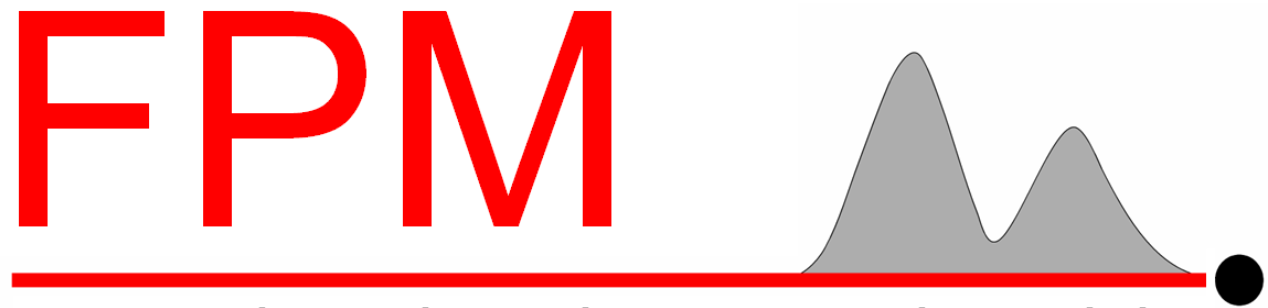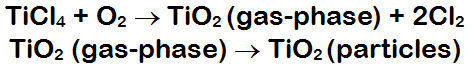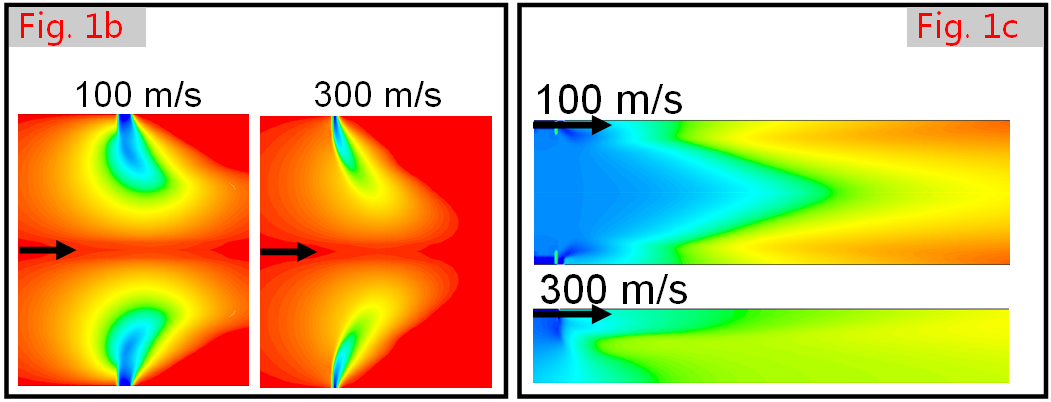Sample Simulation: TiO Particle Formation
The FPM is used to simulate particle formation and growth in
chemical reactors, such as TiO2 particles, according to the
following process:The production of gas-phase TiO2 is simulated with FLUENT, and the
production of TiO2 particles is simulated with the FPM.
.The FPM can be used to simulate the effect of reactor geometry and
operating conditions on the formation of particles. For the reactor
geometry and operating conditions shown in Fig. 1a, and for a given mass
flowrate of TiCl4, the FPM can be used, for example, to simulate the effect
of the velocity of the TiCl4 inlet stream on the radial uniformity of particle
size. Figure 1b shows radial velocity contours for TiCl4 inlet velocities of
100 and 300 m/s, where blue and red indicate the maximum
and minimum radial velocities, respectively. Figure 1c shows contours of
diameter, where blue and red represent minimum and maximum particle
diameters, respectively. Figure 1c shows that the radial distribution of
particle diameter is more uniform for the TiCl4 inlet velocity of 300 m/s
than for 100 m/s. The FPM can also be used to calculate the distribution of
particle number and mass.